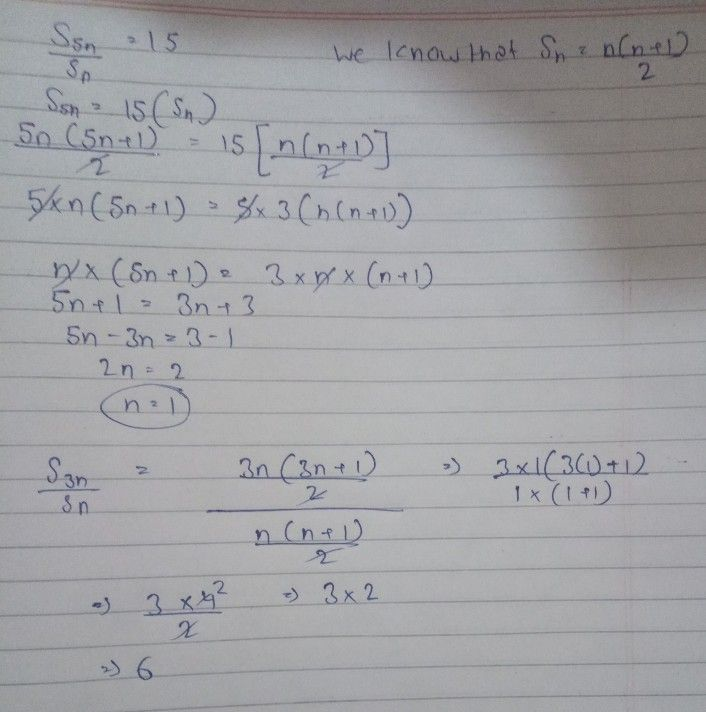Symbol
ProblemLet $S_{n}$ denotes the sum of first $0$ terms of an $A.P$ If $\dfrac {S_{5n}} {S_{n}}=15,$ then the value of $\dfrac {S_{3n}} {S_{n}}$ is
10th-13th grade
Other
Search count: 108
SolutionQanda teacher - ChrisStudent
Thanks